GFG App
Open AppBrowser
Continue

# How to Perform Scheffe’s Test in Excel?

A post hoc test used in the Analysis of Variance is the Scheffe Test, which is also known as Scheffe’s technique or Scheffe’s approach. It carries Henry Scheffe’s name, an American statistician. Scheffe’s test is used to determine whether pairs of means are significant after you have done an ANOVA and obtained a significant F-statistic (i.e., you have rejected the null hypothesis that the means are the same). The Scheffe test adjusts alpha for both straightforward and complex mean comparisons. Multiple pairs of means are compared at once in complex mean comparisons. You can use Fisher’s LSD or Tukey’s HSD, which are the other two mean comparison tests. The Scheffe test has the lowest statistical power but is also the most adaptable. What comparisons you’re interested in determining which test to run:

• Run the Tukey procedure since it will have a narrower confidence interval if you simply wish to compare pairs of data.
• Run the Scheffe test since it will have a narrower confidence interval if you want to compare all potential simple and complex pairs of means.

## Scheffe’s Test

A one-way ANOVA is used to determine whether there is a statistically significant difference between the means of three or more independent groups. We have sufficient proof that at least one of the group means differs from the others if the total p-value from the ANOVA table is less than a certain level of significance. This does not, however, indicate which groups differ from one another. It merely informs us that not all group ways are created equal. We need to perform a post-hoc test that can regulate the family-wise error rate to determine precisely which groups differ from one another. Scheffe’s test is one of the most often applied post hoc tests.

### How to Perform Scheffe’s Test in Excel

Step 1: Enter the Data.

Step 2: Conduct a One-Way ANOVA.

Step 3: Carry out the Scheffe Test.

## Steps to Scheffe Test in Excel

Step 1: First, enter the data. Consider a scenario where a company is trying to determine whether or not three alternative marketing strategies affect salesmen’s performance differently. They randomly assign 10 salesmen to each marketing strategy to test this, and they track their sales results. First, we’ll enter the sales for each salesperson according to the chosen marketing strategy: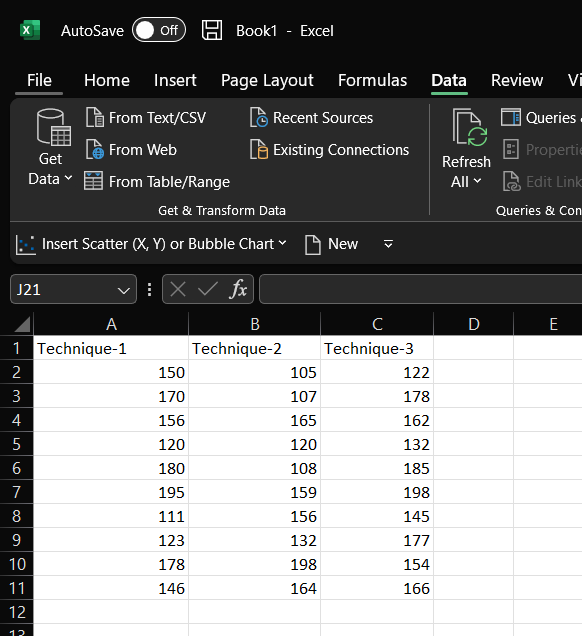Step 2: Perform a One-Way ANOVA in step two. Click the Data tab in the top ribbon, then select the Data Analysis option under the Analysis group to carry out a one-way ANOVA. ( if the option is available)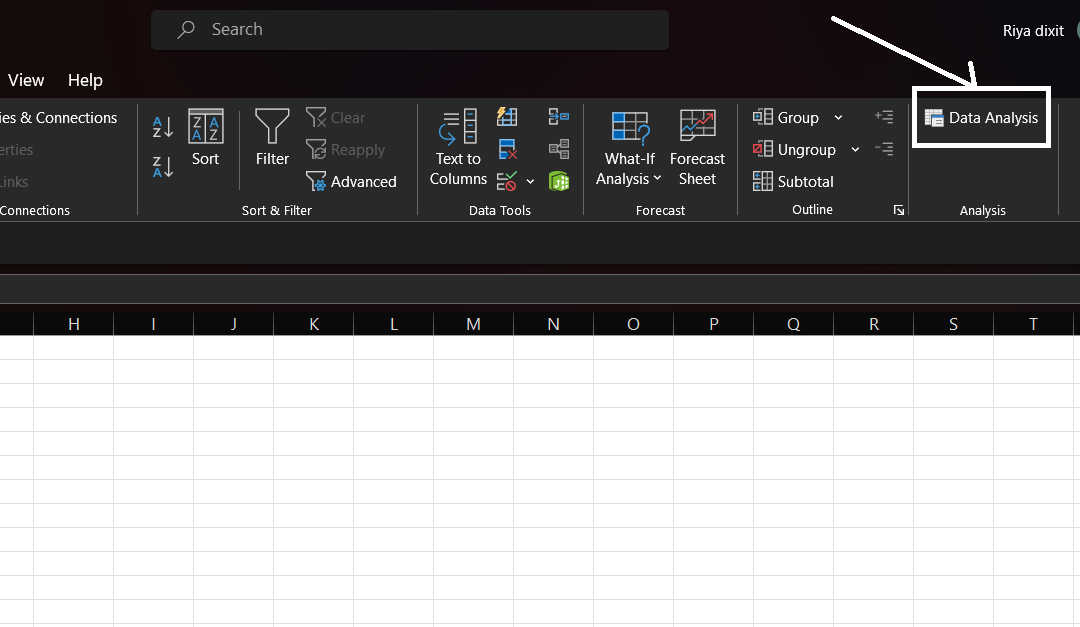If this option isn’t available, you must first get the Analysis ToolPak. Go to files and then choose options to do this.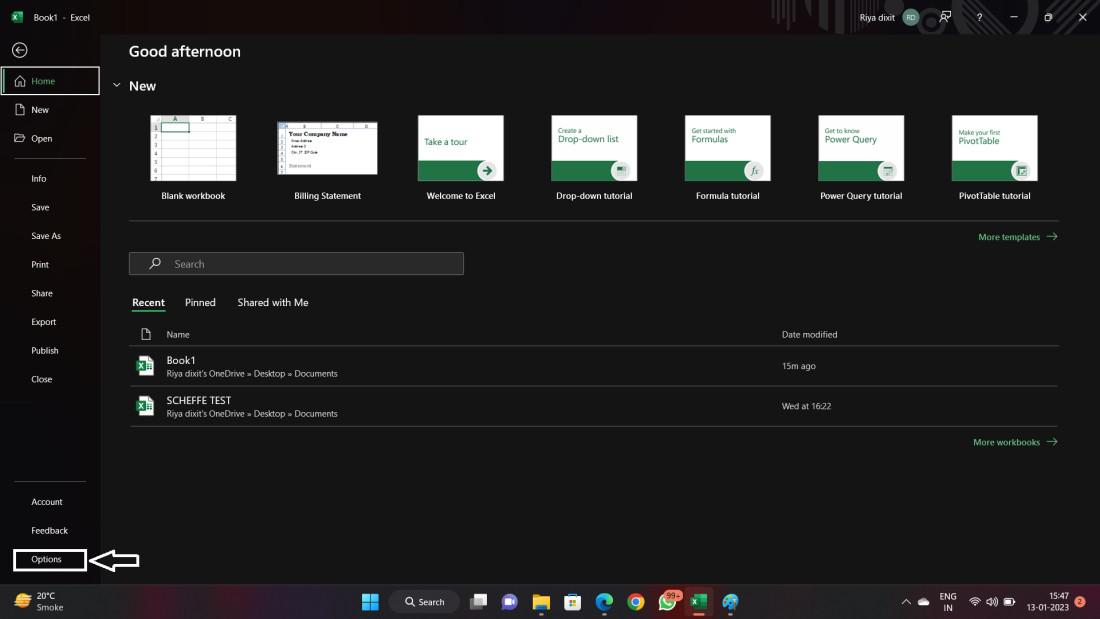Step 3: You will see the options that are shown below in the image and go to option Add-ins.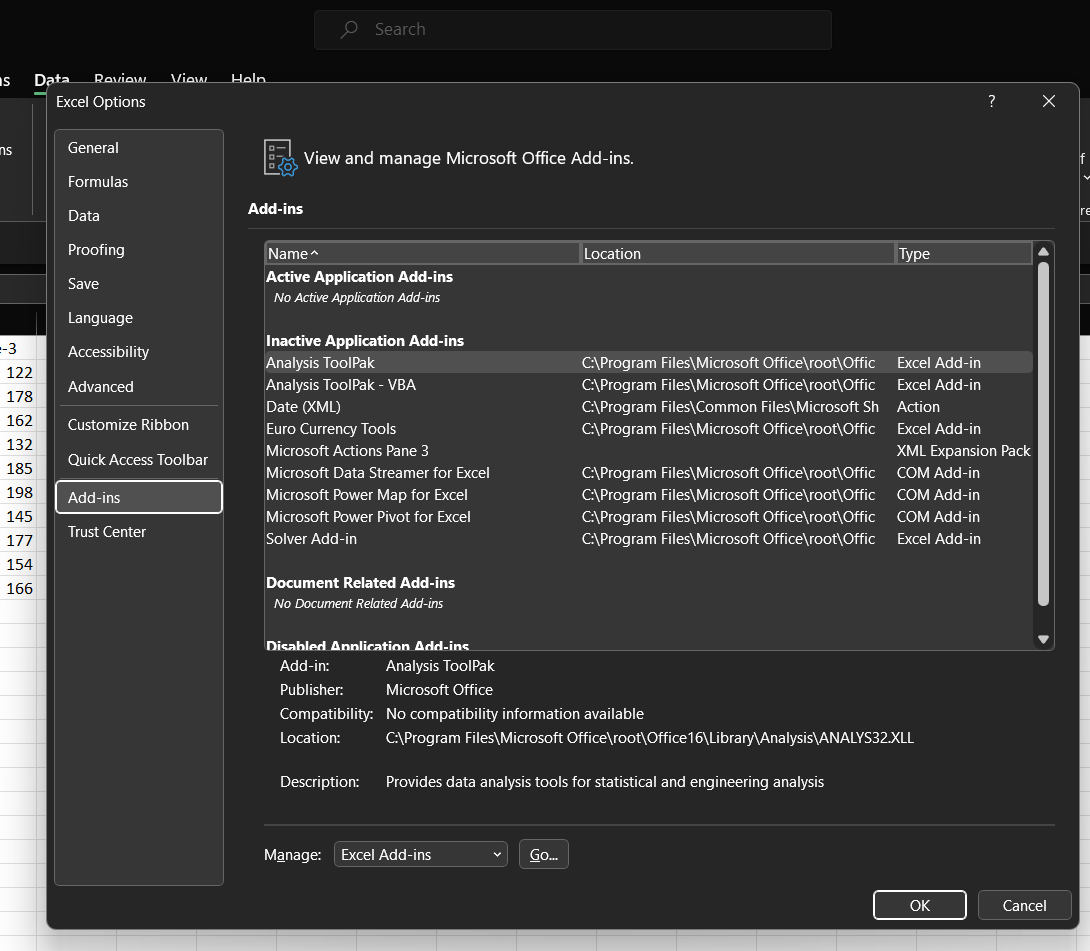Step 4: Then select the option Analysis ToolPak.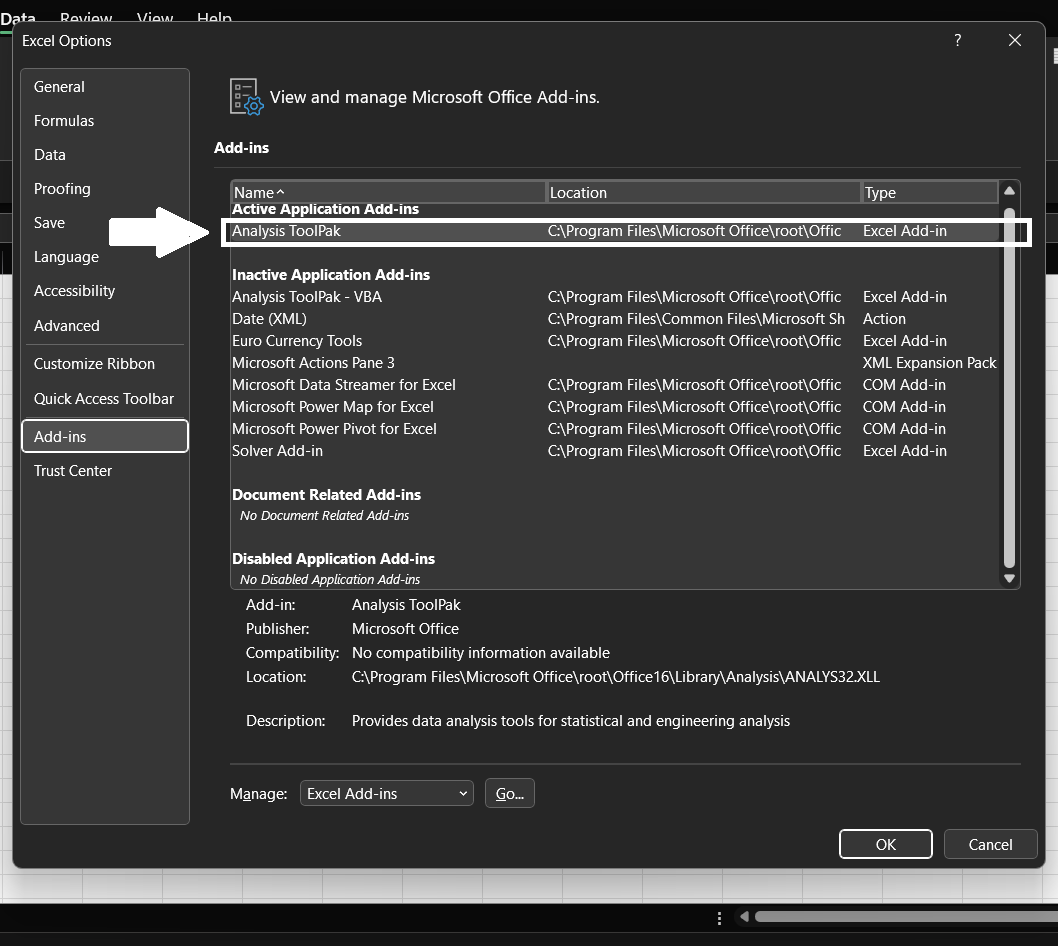Step 5: Now choose the option that is shown below in the picture.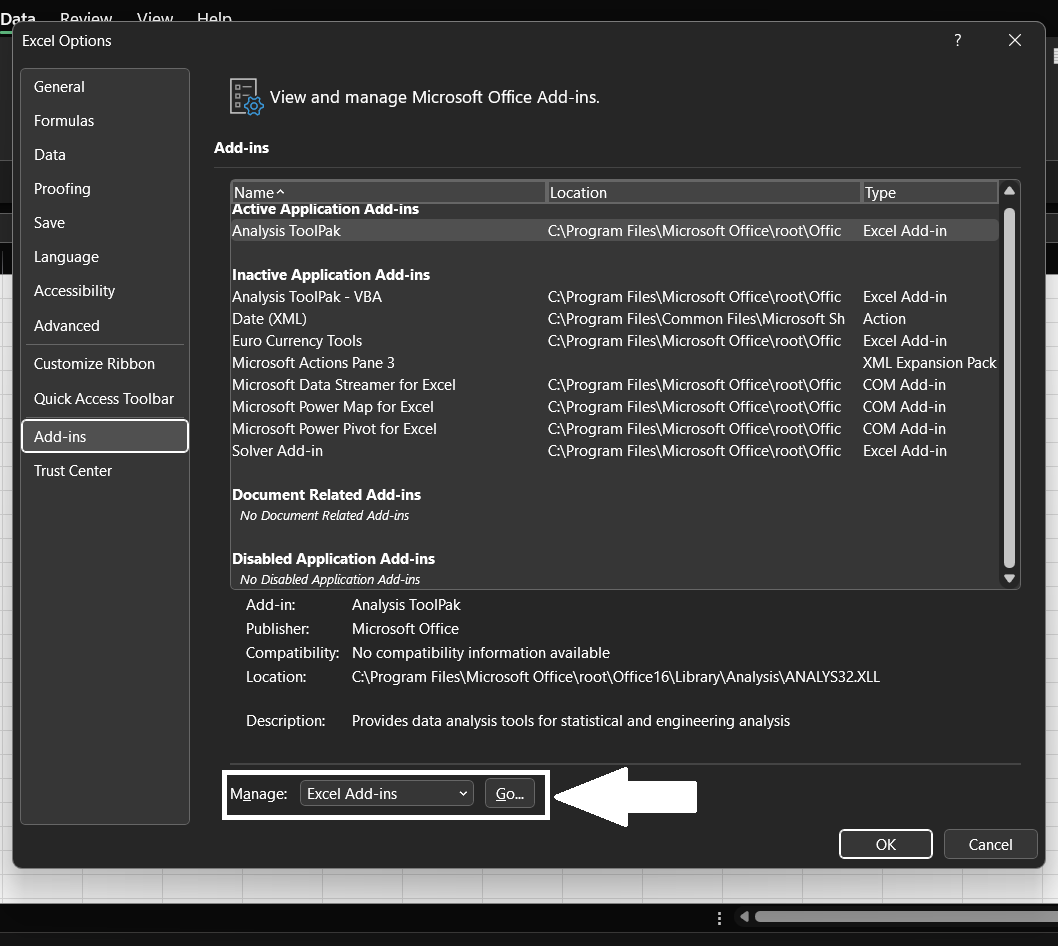Step 6: Now here you will see options and have to select Analysis ToolPak and then click ok.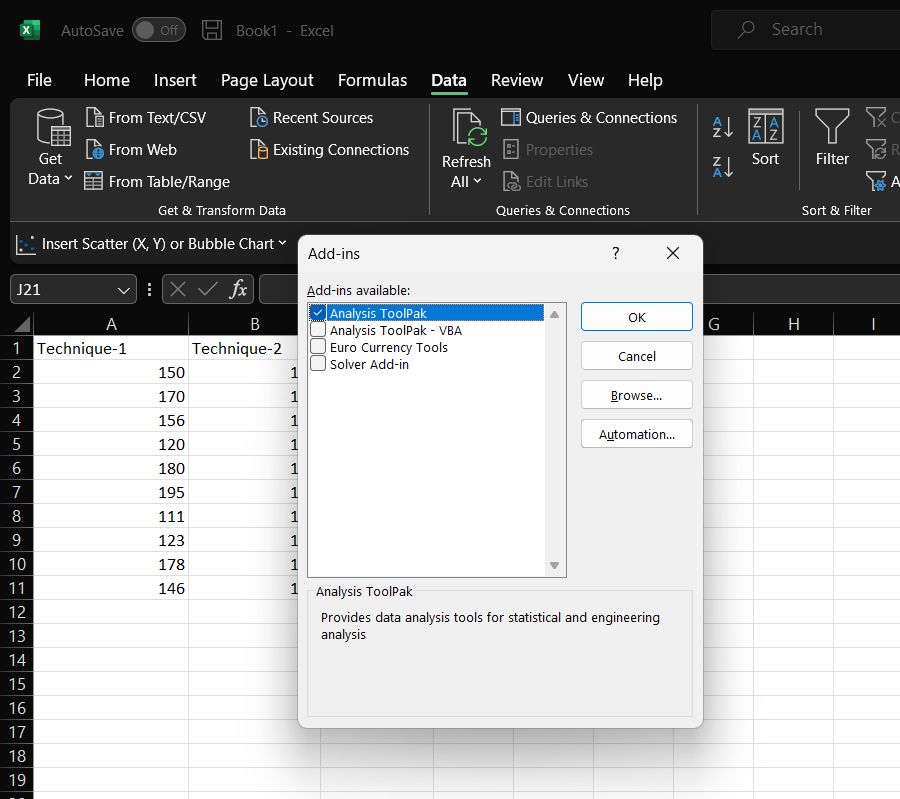Step 7: Now you will observe here data analysis in excel.Step 8: Then select Data Analysis from the list of options. Select the option Anova: Single Factor, and then select OK.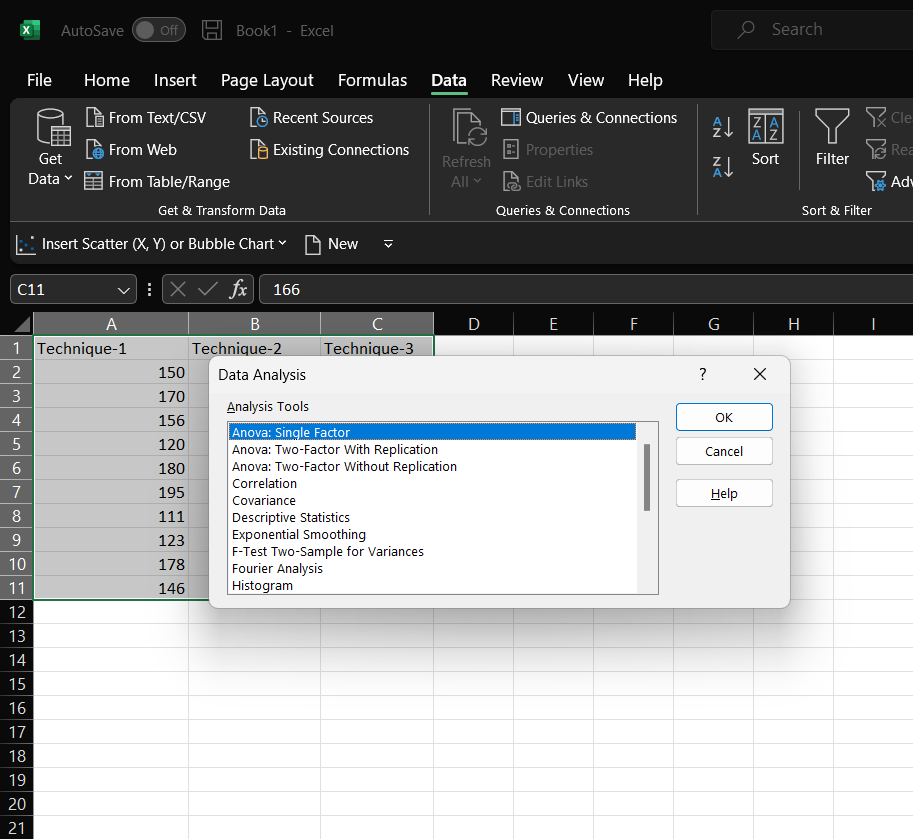Step 9: Fill out the following information in the newly shown window,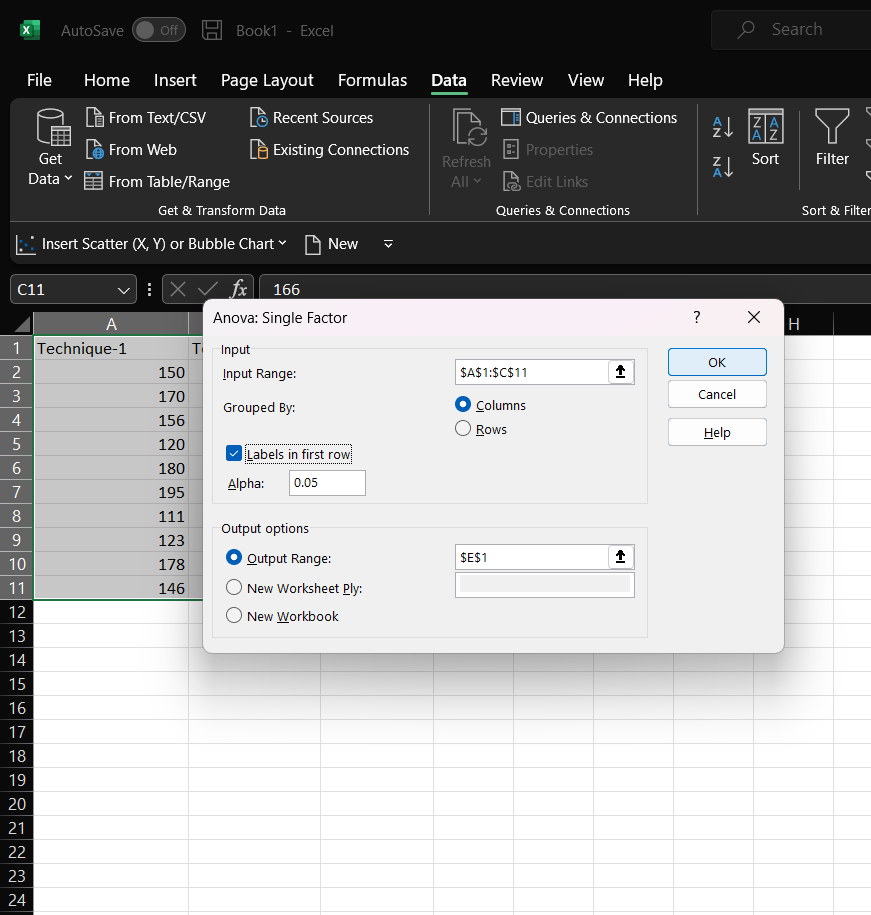filling information

Step 10: The one-way ANOVA results will show up after you click OK.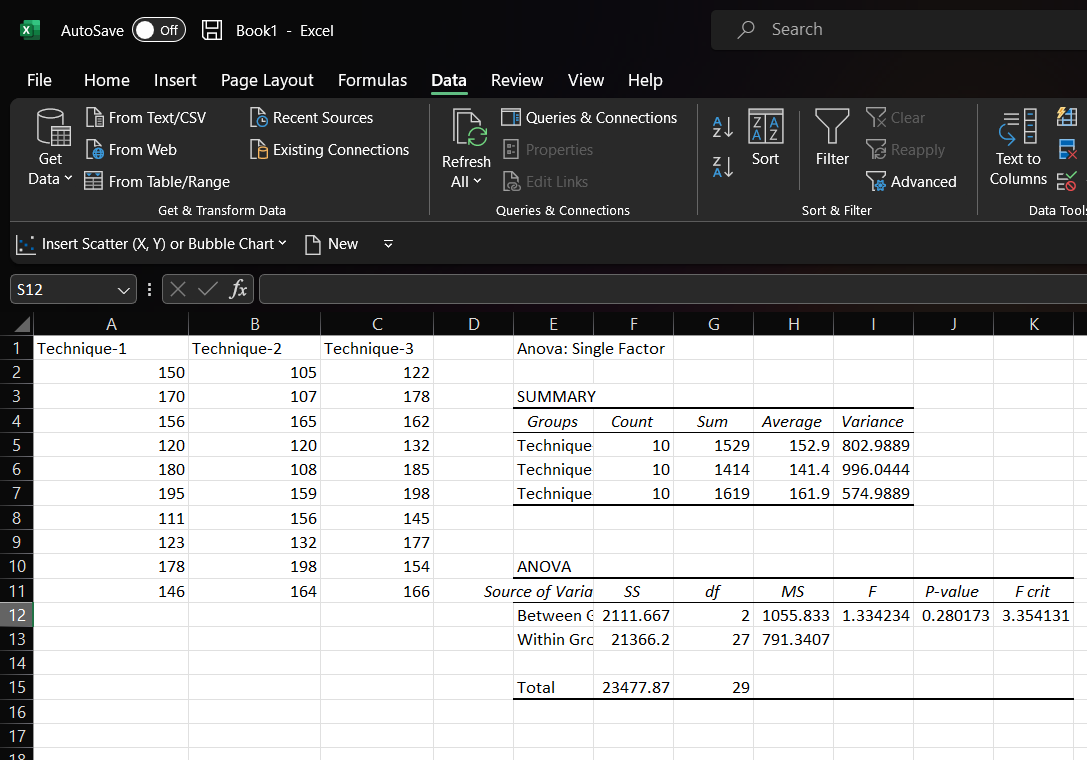Since each group does not have the same average sales score, the overall p-value (0.280173) in the ANOVA table is less than.05. Scheffe’s test will then be used to identify the groups that vary.

Step 11: Performing the Scheffe’s Test. We must first determine Scheffe’s essential value. This is determined as, Scheffe’s Critical Value can be determined through the formula of F-Critical Value * 2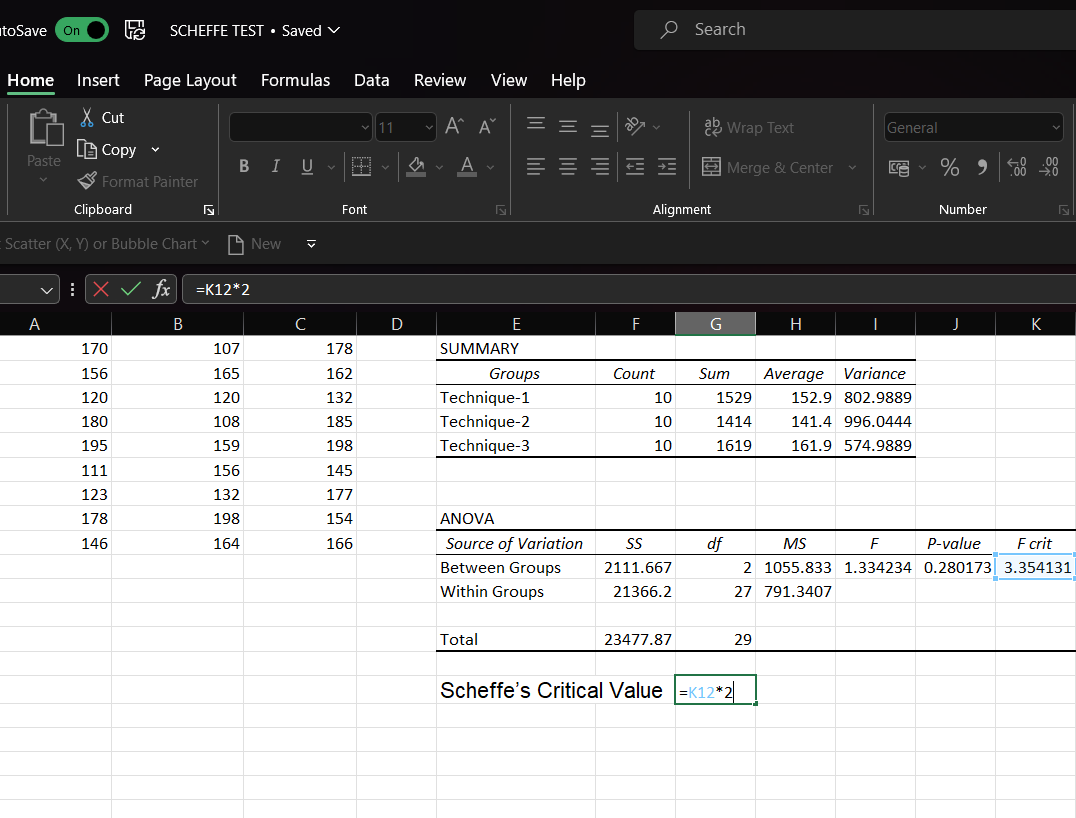Step 12: After entering a formula, press enter and you will get your resultant value.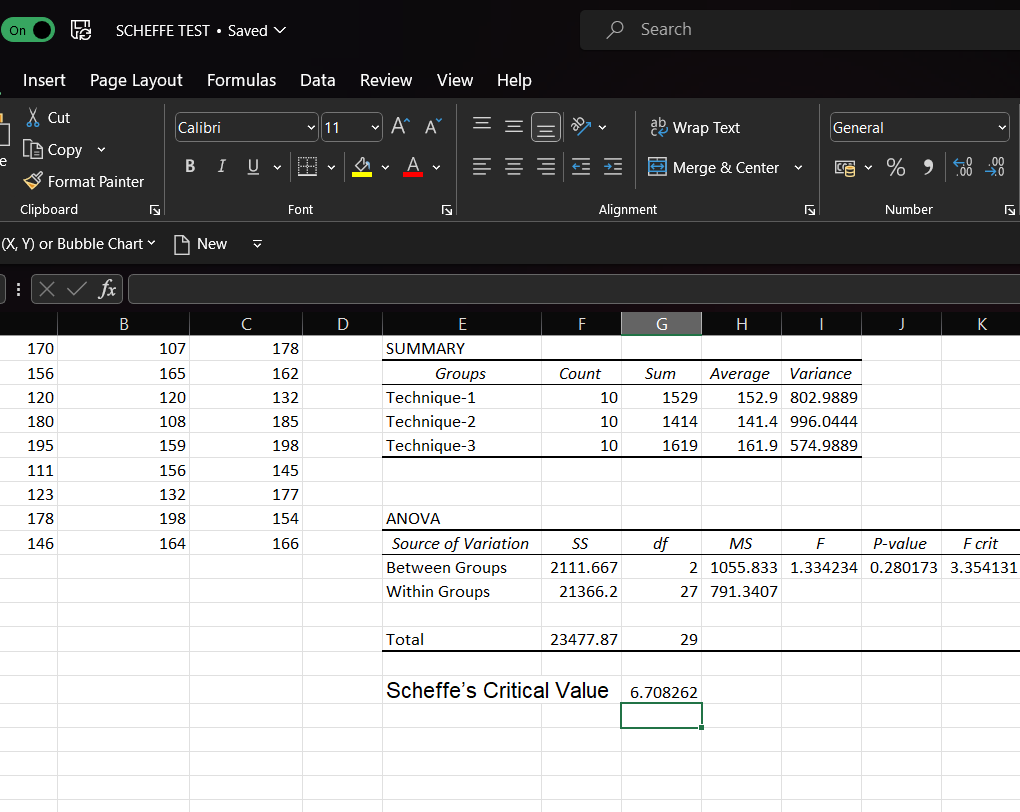Step 13: Next, The F-statistic for each pairwise comparison is then calculated as follows:

F-statistic: (x1–x2)2/(MS within(1/n1 + 1/n2))

For example, we can compute the F-statistic for the pairwise difference between each technique using the formulas below: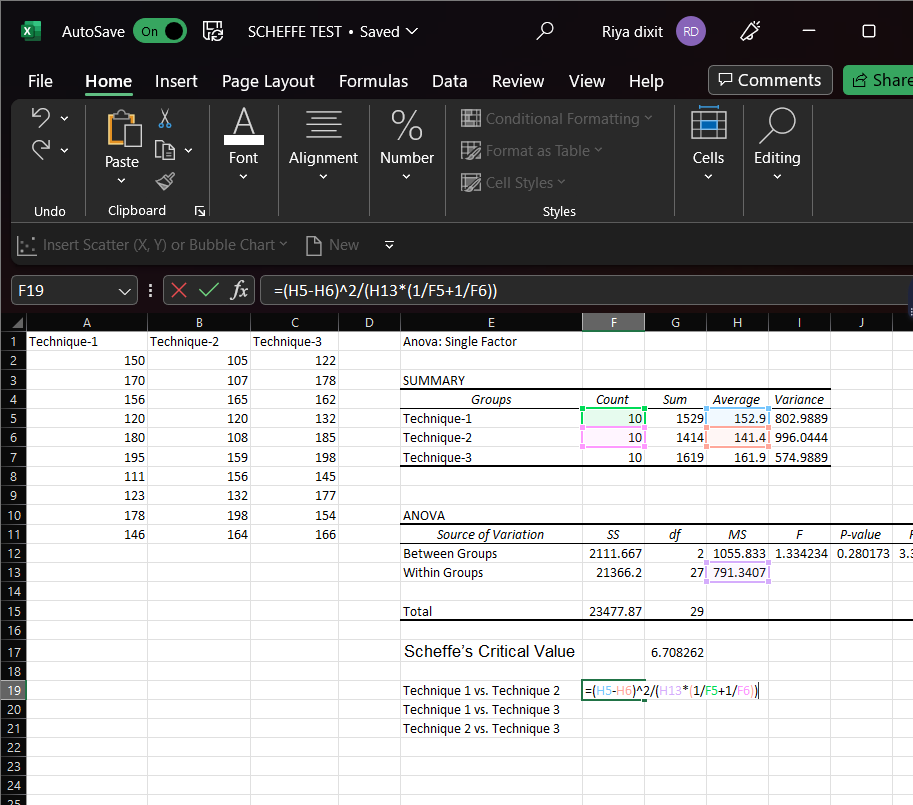Step 14: Enter the formula and then click enter and you will get your resultant value.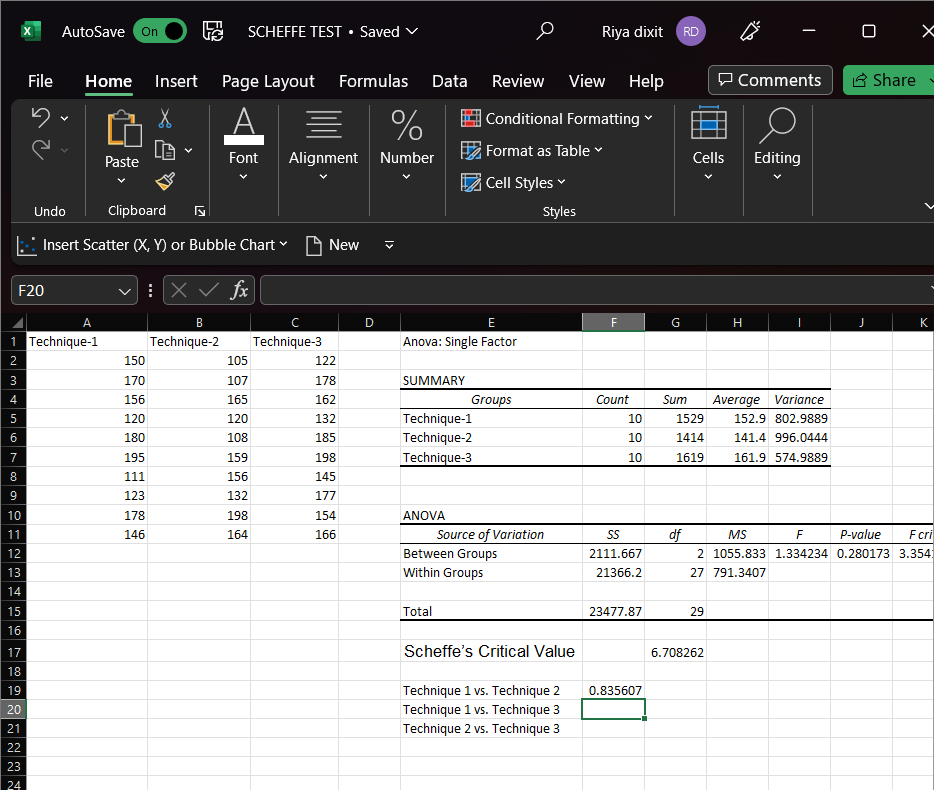Step 15: Enter the formula and then click enter and you will get your resultant value.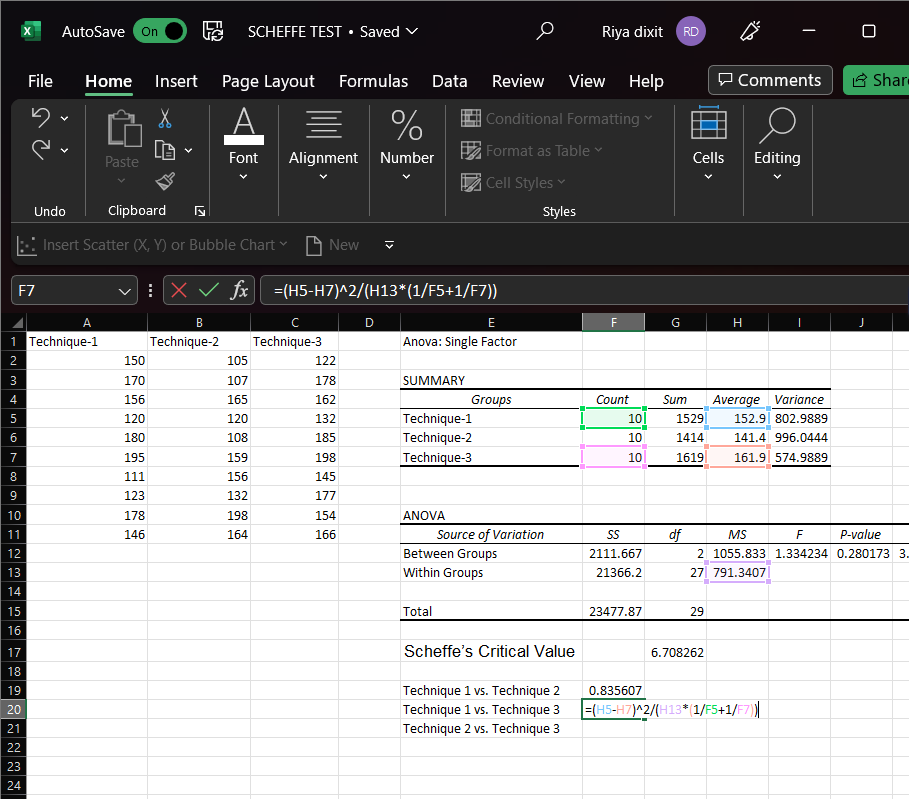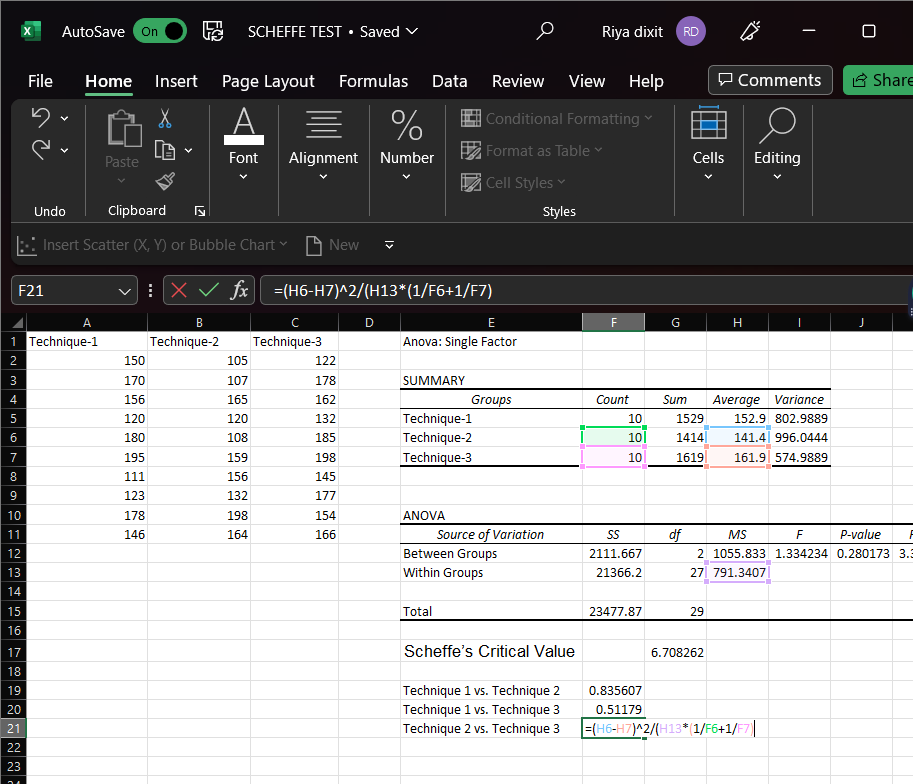Enter the formula and then click enter and you will get your resultant value.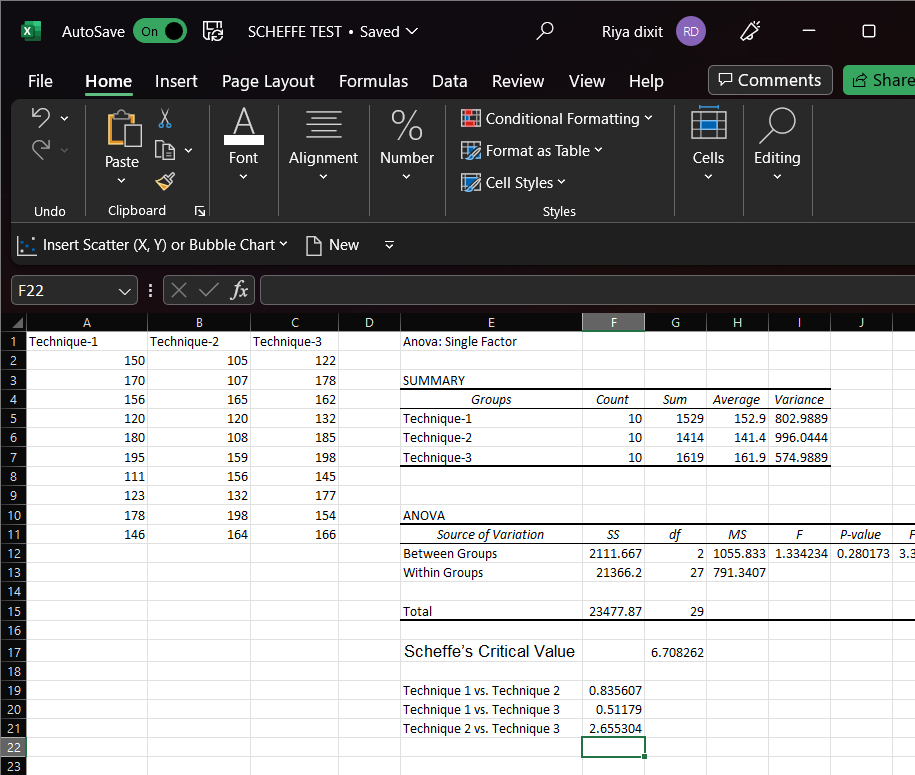No one has an F-statistic greater than Scheffe’s Critical Value. Consequently, the no groups differ statistically significantly.

My Personal Notes arrow_drop_up